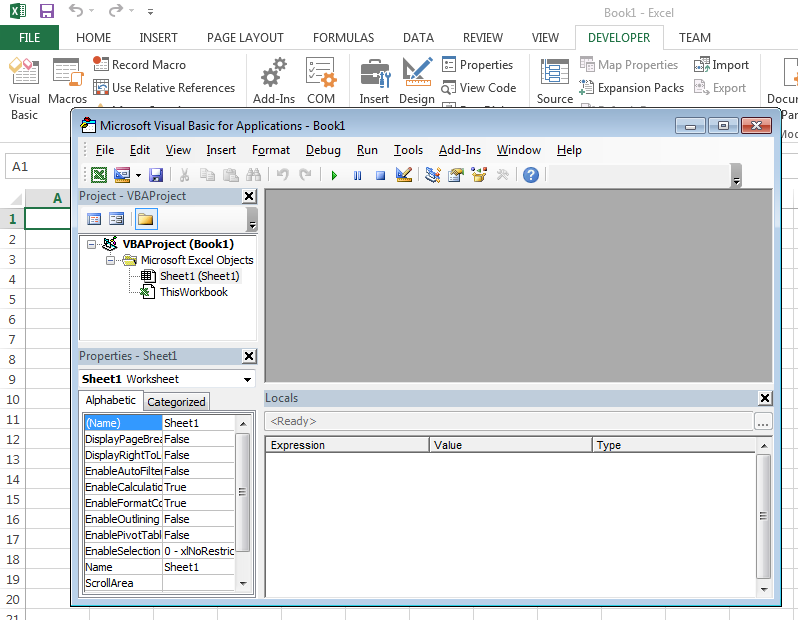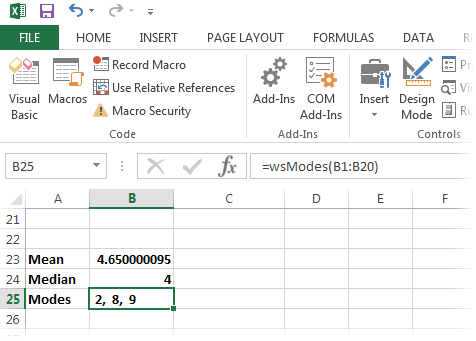# Calling Fortran DLLs from Excel

Posted on 2017-07-26

In the last blog post, we created a simple dynamic link library for Windows that was easily callable from C, including Microsoft's Visual C++. As stated in the article, the resulting DLL was entirely standards-compliant: it utilized proper calling conventions and it exported the functions we wished to expose. Therefore, we can take another step and call said DLL from Microsoft Office Excel.

Excel spreadsheets are often used for data analysis, and, occasionally, when non-trivial analyses are necessary, Fortran may make life a bit easier. Our Fortran DLL contained three functions for computing three types of averages, but countless, more complicated use cases exist. To keep this explanation simple, though, we'll proceed with interfacing with our averaging procedures.

A Fortran DLL, or any DLL for that manner, isn't directly interfaced with Excel. We first need a small amount of glue in the form of Visual Basic for Applications, or VBA, code. This BASIC code is necessary to define the interface to the DLL, transform the input data properly, and expose functions to the Excel worksheet. To get started on writing this glue, we need to open Excel's VBA editor, accessible either with the Alt-F11 hotkey or clicking "Visual Basic" under the Developer menu. This should open the Visual Basic for Applications editor:Next, we'll need a new "Module" for our routines, which can be added by selecting "Module" from the Insert menu. This option should present a BASIC editor for defining our routines.

The first step is to create prototype calls for our Fortran subroutines. Recall our definition of the mean subroutine was:

``````      function mean(xc, n) bind(c)
use ISO_C_BINDING
implicit none
cGCC\$ ATTRIBUTES STDCALL, DLLEXPORT :: mean

real(kind=c_float)::mean

type(c_ptr), value::xc
integer(kind=c_int), value::n
``````

The equivalent VBA declaration would be:

``````Private Declare PtrSafe Function mean Lib "C:\workspace\ExcelDll\fortran-library.dll" _
(ByRef x As Single, ByVal n As Integer) As Single
``````

The above prototype has a few details that require explaining. First, we've declared this function with the "PtrSafe" attribute as required by 64-bit Excel; if a user is running 32-bit Excel, this attribute should not be included. Second, the prototype includes the full path to the DLL in question, and this path should be changed appropriately. Third, our array, x in the VBA prototype and xc in the Fortran function declaration, is not declared as an array in VBA. Rather, when using the "ByRef" attribute, we're effectively passing the memory address of the first element of the array. Subsequent elements are assumed to be stored immediately after the first in a true C-compatible, one-dimensional array, so the above is sufficient. Visual Basic for Applications treats arrays somewhat differently than C does, so we're not using a true VBA array as a parameter.

Our remaining Fortran functions are prototyped in VBA as:

``````Private Declare PtrSafe Function median Lib "C:\workspace\ExcelDll\fortran-library.dll" _
(ByRef x As Single, ByVal n As Integer) As Single

Private Declare PtrSafe Function mode Lib "C:\workspace\ExcelDll\fortran-library.dll" _
(ByRef x As Single, ByVal n As Integer, ByRef y As Single) As Integer
``````

To use our functions on a spreadsheet, we need to create some VBA functions to call these routines properly and format the data accordingly. Taking the mean function as our initial example, we would want to define a worksheet-callable function, wsMean, as such in VBA:

``````Function wsMean(x As range) As Single
Dim n As Integer
Dim p() As Single

p = Range2Array(x, n)

wsMean = mean(p(1), n)

End Function
``````

A worksheet function for computing the mean will accept a range of cells and return a single mean value for these given cells. The first step, though, is to convert the range of cells to a true VBA array. In our case, we can write a small, reusuable function that accepts a range and returns a one-dimensional array of values, Range2Array:

``````Function Range2Array(x As range, n As Integer)
Dim res() As Single
n = x.Rows.Count

ReDim res(1 To n)

For irow = 1 To n
res(irow) = x.Cells(irow, 1).Value
Next irow

Range2Array = res
End Function
``````

Much of the code above is specific to Excel and VBA, but it is basically reading the value of each cell and assigning each cell's numeric value to an element of a properly-sized array. One might note that this routine only translates a single column. The user, though, can quickly extend this to multiple columns.

Returning to our wsMean wrapper, the call to the Fortran mean routine was:

``````wsMean = mean(p(1), n)
``````

We appear to be passing only the first element of the array. However, recall that the function definition actually declared this argument as "ByRef," meaning that VBA should pass the address of this argument, which is also the address of our entire array p. Therefore, our Fortran routine will receive the equivalent of a C pointer to a block of memory containing our one-dimensional array.

The median function can be wrapped accordingly:

``````Function wsMedian(x As range) As Single
Dim n As Integer
Dim p() As Single

p = Range2Array(x, n)

wsMedian = median(p(1), n)

End Function
``````

Note that wsMedian is written nearly identical to wsMean since they both accept an array and a length, and return a single number. Our Fortran mode function is slightly more complex since it returns results in an array passed as an argument. The wrapper wsModes appears below:

``````Function wsModes(x As range) As String
Dim n As Integer
Dim p() As Single

Dim res() As Single
Dim resString() As String
Dim rescount As Integer

p = Range2Array(x, n)

ReDim res(1 To n)

rescount = mode(p(1), n, res(1))

If (rescount = 0) Then
wsModes = "None"
Else
ReDim resString(1 To rescount)
For i = 1 To rescount
resString(i) = Str(res(i))
Next i

wsModes = Join(resString, ", ")
End If

End Function
``````

In wsModes we need to define a results array to pass to our Fortran function. If the Fortran mode function returns a result of zero modes, this function will actually populate a cell with the string "None." If valid modes are returned, a text list of modes is created to populate a single cell.

Our three worksheet functions, wsMean, wsMedian, and wsModes, are now all callable directly from an Excel cell:The resulting spreadsheet can now indirectly call our Fortran procedures, passing data from spreadsheet cells and properly populating resulting cells.

For time-consuming calculations, this exposure of Fortran procedures to the worksheet itself might be a poor choice because Excel often recomputes sheets when values change. The user might be better off inserting a button that triggers calls to Excel in those cases to limit the amount of computing necessary to refresh any given worksheet.

A macro-enabled spreadsheet along with the original Fortran DLL code is attached below:

The VBA code in the Excel spreadsheet will need to be updated with the proper path to the Fortran DLL since it currently refers to a specific, absolute path on our demonstration system. Once updated, though, Excel should be able to interface with a Fortran DLL quite easily.

Back to the Blog...